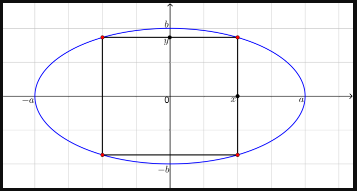# Find the area of the largest rectangle that can be inscribed in the ellipse x^2/a^2 + y^2/b^2 = 1

Find the area of the largest rectangle that can be inscribed in the ellipse x^2/a^2 + y^2/b^2 = 1